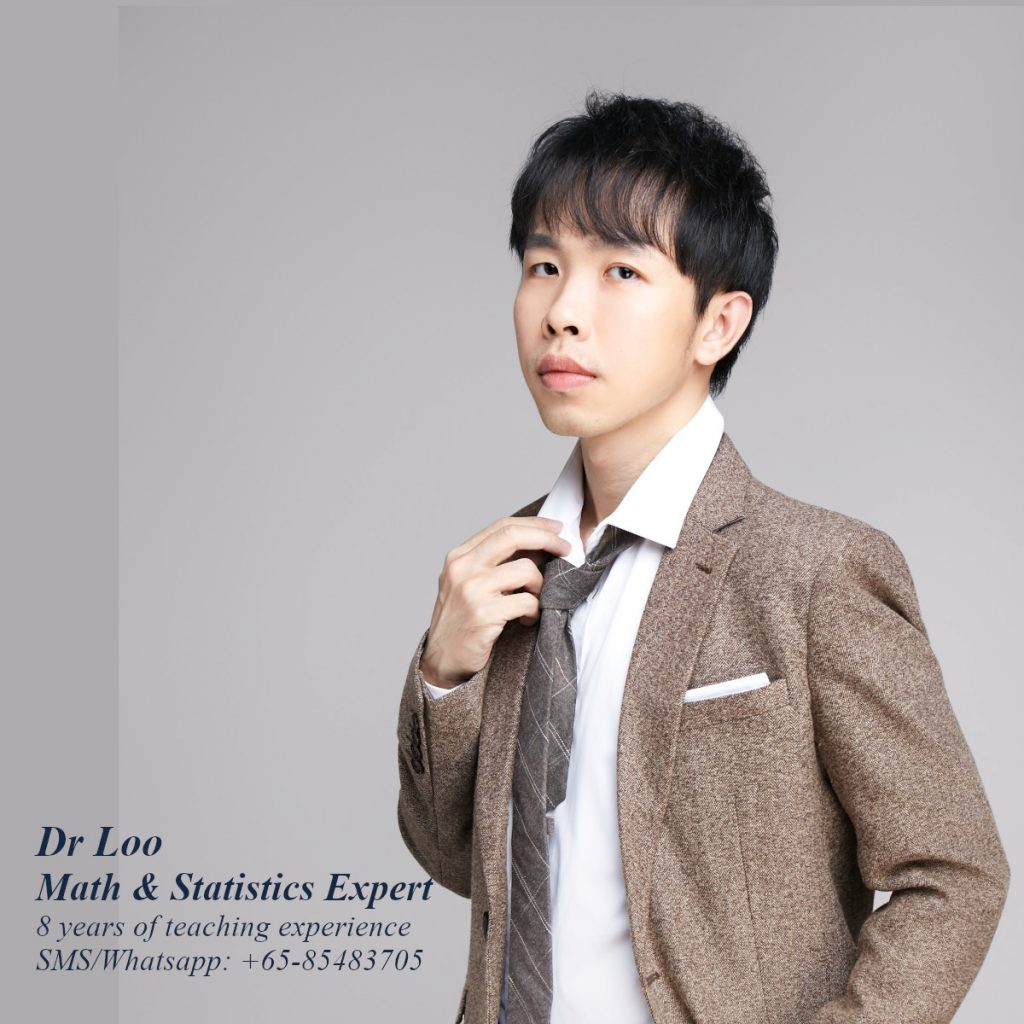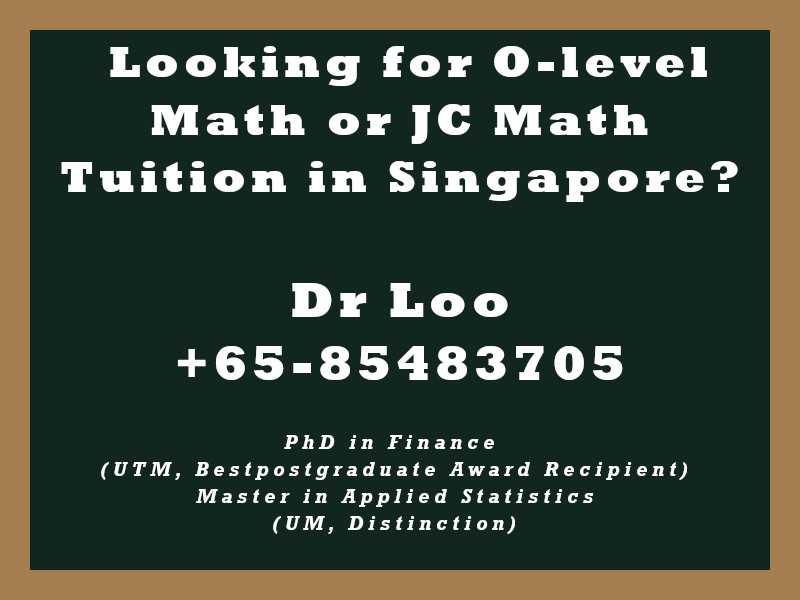+65-85483705
Select Page

# Correlation

Purpose – To measure the relationship between two variables. Students will normally learn the formula in calculating correlation in A-level mathematics class. However, the basic concept on the correlation which is the scatter plot maybe be introduce during the O-level mathematics lessons.

## Formula

Correlation = Covariance of the two variables, x & y and divide it by the standard deviation of x and standard deviation of y.

## How to determine the siginificance of the correlation value

Taking the sample size, n, then minus it with 2 in order to obtain the degree of freedom in order to obtain the critical value from the statistics table. df = n – 2.

Question

Calcuate the correlation values for the following set of the variables.

1. The correlation between Variable 1 & Variable 2.

 variable 1 variable 2 1 4 1 4 4 2 3 4 5 1 4 2 1 2 0 0 1 1 5 3 4 2 4 4 5 5 0 4

2. The correlation between Variable 3 & Variable 4.

 variable 3 variable 4 4 4 5 2 1 1 1 2 5 0 0 0 5 2 1 3 5 0 1 2 5 4 5 3 3 4 2 2

3. The correlation between Variable 5 & Variable 6.

 variable 5 variable 6 1 1 5 5 3 3 3 4 2 3 5 1 3 2 2 1 2 5 5 3 4 4 4 4 4 5 4 5

4. The correlation between Variable 7 & Variable 8.

 variable 7 variable 8 5 2 5 4 3 0 1 5 0 2 3 5 2 3 5 2 2 5 1 2 5 1 2 5 0 5 2 1

5. The correlation between variable 9 & variable 10.

 variable 9 variable 10 5 1 1 5 1 1 4 4 3 4 0 0 3 2 4 2 5 1 0 2 3 4 4 3 2 3 4 5

1. Correlation between variable 1 & variable 2 = 0.129
2. Correlation between variable 3 & variable 4 = 0.16
3. Correlation between variable 5 & variable 6 = 0.335
4. Correlation between variable 7 & variable 8 = -0.304
5. Correlation between variable 9 & variable 10 = 0.138

## About the Math Tutor - Dr Loo

I am a PhD holder with 9 years of teaching experience at secondary school and university. My expertizes are mathematics, statistics, econometrics, finance and machine learning.

Currently I do provide consultation and private tuition in Singapore. For those who are looking for math tutor in Singapore or statistics tutor in Singapore, please feel free to contact me at +65-85483705 (SMS/Whatsapp/Telegram).

Some of the math tuition in Singapore I provide includes O-level math tuition and also JC Math Tuition (H1 Math Tuition & H2 Math Tuition). On the other hand, my statistics tuition in Singapore mostly focus on pre-university level until postgraduate level. For those who prefer online tutoring service, the online O-level math tuition and online JC math tuition are also available.## For those who are looking for math tution in Singapore

Need help with this topic? I do provide mathematics home tuition in Singapore for O-level math and also JC H2 math. In addition, online math tutoring is available as well. Feel free to contact me if you would like to know further.